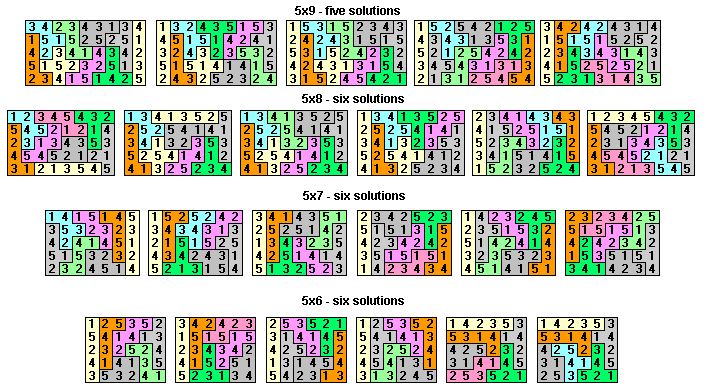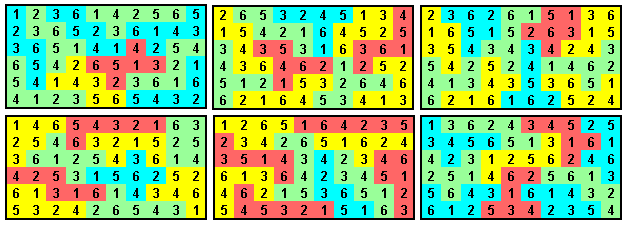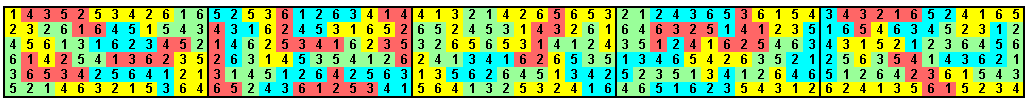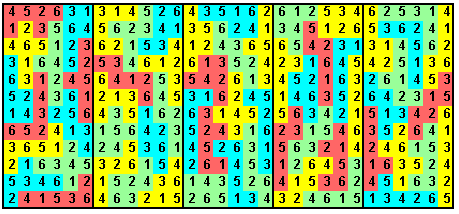Numbered Polyominoes

Given a solution to the 5x12 rectangle with pentominoes, number each square so that

(a) each pentomino contains the numbers 1 to 5
(b) each column contains the numbers 1 to 5
(c) the numbers in each row total 36
(d) no two equal numbers are adjacent horizontally

William Rex Marshall has solved this problem for all 1010 solutions to the 5x12 rectangle. Fifty solutions are shown here.We could also look at 5xn rectangles with an extra condition, viz

(e) no equal numbers can join diagonally.

In this case neither the U nor the V pentomino can be included in a solution

William Rex Marshall has solved this problem also. There are no solutios for n=10 or n<6. The remainig solutions are shown here.We can also look at the hexominoes for similar problems. The diagram below shows a 6x60 rectangle made with a full set of one-sided hexominoes in which

(a) each hexomino contains the numbers 1 to 6
(b) each column contains the numbers 1 to 6
(c) the numbers in each row total 210
(d) no two equal numbers are adjacent horizontallyAgaian a number of pieces will not allow condition (e) above to be satisfied, but an improvemnet would be a solution in which (c) were replaced by -

(c) each of the numbers 1 to 6 occurs ten times in each row.

This set can also be made into six 6x10 rectangles with each row adding to 35. Note that if the bottom row is added to the right of the top row we get a solution to the above problem.Condition (c) above can be achieved, the solution below goes further in having five 6x12 rectangles with each nember appearing twice in each row of each rectangle. With minor changes of numbers, these five can also be joined to fram a 12x30 rectangle.The type of problem above lends itself to forming Sudoku type problems. Some examples are here.